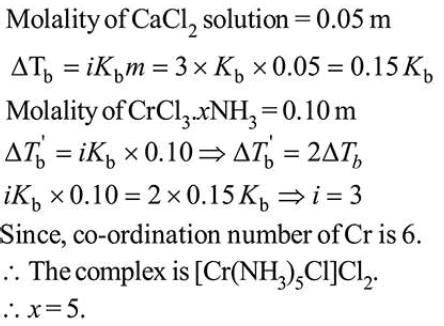# The elevation of boiling point of 0.10 m aqueousQuestion:

The elevation of boiling point of $0.10 \mathrm{~m}$ aqueous $\mathrm{CrCl}_{3} \cdot x \mathrm{NH}_{3}$ solution is two times that of $0.05 \mathrm{~m}$ aqueous $\mathrm{CaCl}_{2}$ solution. The value of $x$ is____________________

[Assume $100 \%$ ionisation of the complex and $\mathrm{CaCl}_{2}$, coordination number of $\mathrm{Cr}$ as 6 , and that all $\mathrm{NH}_{3}$ molecules are present inside the coordination sphere]

Solution:

(5.0)